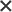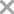0 / 300

## ￥68   体检结果报告如图 能够解读一下要点 老人有高血压和冠心病 消融手术失败 以往检查伴有脂肪肝暂无内容

|

•妇科

•男科

•耳鼻咽喉科

•皮肤科

• 骨科
• 儿科
• 妇产科
• 口腔科
• 神经内科
• 泌尿外科
• 神经外科
• 乳腺外科
• 眼科
• 普外科
• 感染科
• 整形外科
• 外科
• 心血管内科
• 消化内科
• 呼吸内科

•柏愚 副主任医师 在线

上海长海医院 消化内科

• 直肠息肉
• 出血
• 急性胃肠炎
• 慢性浅表性胃炎
• 结肠炎
• 急性化脓性胆囊炎
• 食管贲门黏膜撕裂综合征
• 慢性胰腺炎急性发作
• 胃食管反流病
• 肠息肉
• 胰石症
• 急性糜烂性胃炎
• 肠易激综合症
• 胃潴留
• 胆囊癌切除个人史
• 胰管扩张
• 胆囊息肉
• 胆囊切除术后
• 溃疡性结肠炎
• 肠炎
• 胃癌前病变
• 十二指肠球部炎
• 反流性食管炎
• 萎缩性胃炎
• 肝硬化
• 胰管结石
• 慢性萎缩性胃炎
• 慢性胰腺炎
• 胃炎
• 食管炎
• 结肠息肉
• 胆囊结石
• 慢性胃炎
• 胰腺癌
• 胃体恶性肿瘤
• 小儿炎症性肠病
• 肝胆管结石
• 胃病
• 急性肠胃炎
• 急性胰腺炎
• 胆总管结石
• 食管平滑肌瘤
• 胰腺炎
• 消化不良
• 胃息肉
• 胃溃疡
• 便秘
• 功能性消化不良
• 肝囊肿
• 胆囊炎
• 消化道出血
• 胰腺囊肿
• 胆道狭窄
• 胰腺异位
• 食管癌
• 呼吸道感染
• 幽门螺杆菌感染
• 巴雷特食管
• 胆管结石
• 胃肠功能紊乱
• 十二指肠溃疡

759个回答 ·100%回答率 ￥898 问TA

•代杰文 副主任医师 在线

上海交通大学医学院附属第九人民医院（南部院区） 口腔颅颌面科

• 口腔疾病
• 牙瘤
• 牙痛病
• 牙折断
• 下颌第三磨牙冠周炎
• 口腔牙体病
• 涎腺黏液囊肿
• 牙龈癌
• 牙龈萎缩
• 额外牙
• 唇裂
• 牙体缺失
• 颌面畸形
• 牙周炎
• 牙齿畸形
• 舌乳头炎
• 口呼吸引起的错合
• 感染
• 扁桃体肥大伴有腺样体肥大
• 舌炎
• 偏颌
• 阻生齿
• 牙列不齐
• 牙髓钙化
• 智齿
• 牙髓疾病
• 萎缩性舌炎
• 下颌畸形
• 牙髓炎
• 牙龈瘤
• 牙龈增生
• 慢性唇炎
• 牙科检查
• 牙颌畸形
• 颞颌关节陈旧性脱位
• 口腔扁平苔藓
• 基底细胞腺瘤
• 颌面部骨折
• 前牙反合
• 磨牙症
• 口腔颌面肿瘤
• 下颌骨骨折
• 舌下囊肿
• 唇腭裂
• 口腔颌面部软组织挫裂伤
• 口腔粘膜溃疡
• 腮腺炎
• 口腔粘膜病
• 上颌骨骨折
• 真性牙骨质瘤
• 颞下颌关节紊乱症
• 牙龈囊肿
• 牙周病
• 龋齿
• 牙磨损
• 皮样癌
• 颞下颌关节炎
• 牙龈出血
• 裂沟舌
• 腭裂
• 牙痛
• 慢性根尖周炎
• 颌骨囊肿
• 乳牙滞留
• 口腔溃疡
• 根尖周囊肿
• 颌面部外伤
• 额外牙[多生牙]
• 上颌骨囊肿
• 上颌前突
• 成人牙周炎
• 舌下腺囊肿
• 埋伏牙
• 地图舌
• 下颌后缩
• 腮腺肿瘤
• 乙肝
• 自行摔伤
• 颌骨结核
• 小儿鹅口疮
• 先天性鳃裂囊肿
• 面部血肿
• 口腔创伤性血泡及溃疡
• 深覆颌
• 鹅口疮
• 牙外伤
• 智齿冠周炎
• 多形性腺瘤
• 前牙反颌
• 颌下淋巴结炎
• 慢性化脓性腮腺炎
• 儿童牙颌畸形
• 关节痛
• 颧骨骨折
• 颞颌关节疾病
• 牙槽突裂
• 含牙囊肿
• 口腔颌面部后天畸形和缺损
• 舌白斑
• 根尖囊肿
• 骨折
• 埋伏牙和阻生齿
• 阻生牙
• 下颌骨囊肿
• 急性冠周炎
• 牙龈脓肿
• 口腔炎
• 牙髓坏死
• 牙龈炎

599个回答 ·100%回答率 ￥30 问TA

•邹宸 主治医师 在线

复旦大学附属眼耳鼻喉科医院 眼科

• 眼疲劳
• 先天性眼球震颤
• Stargardt病
• 眼充血
• 泪道梗阻
• 突眼性情况
• 眼痛
• 结膜出血
• 弱视
• 睑腺炎
• 高度近视
• 创伤性面瘫
• 巩膜炎
• 感染
• 老视
• 视神经炎
• 眼黄斑后极变性
• 角膜白斑
• 视网膜病变
• 儿童视力低下
• 玻璃体出血
• 慢性结膜炎
• 鼻泪管阻塞
• 增生性玻璃体视网膜病变
• 眼外伤
• 结膜色素沉着
• 近视
• 结膜炎
• 细菌性结膜炎
• 出血
• 玻璃体疾患
• 角膜炎
• 眨眼症
• 维生素A缺乏合并干眼症
• 球结膜肉芽肿
• 睑板腺炎
• 睑板腺囊肿[霰粒肿]
• 球结膜下出血
• 泪囊炎
• 牵牛花综合征
• 角膜异物
• 结膜异物
• 睑裂斑
• 变应性结膜炎
• 虹膜色素痣
• 老年性白内障
• 间歇性外斜视
• 葡萄膜炎
• 眼皮松弛
• 睑板腺囊肿
• 黄斑疾病
• 维生素A缺乏病
• 视疲劳
• 倒睫
• 视神经病变
• 视力模糊
• 单眼视力下降
• 眼部囊肿
• 高眼压
• 眼球挫伤
• 急性卡他性结膜炎
• 青光眼
• 眼底病
• 复视
• 视网膜病
• 角膜结膜炎
• 干眼病[结膜干燥]
• 瞳孔散大
• 急性眼眶蜂窝织炎
• 散光
• 新生儿睑缘炎
• 泪道阻塞
• 屈光不正
• 眼炎
• 远视
• 眼干燥综合征
• 翼状胬肉
• 视网膜中央动脉阻塞
• 眼科瘤病
• 与缺乏休息和空暇时间有关
• 圆锥角膜
• 病毒性结膜炎
• 虹膜睫状体炎
• 斜视性弱视
• 假性近视
• 角膜损伤
• 黄斑水肿
• 异物入目病
• 视网膜中央静脉阻塞

392个回答 ·98%回答率 ￥18 问TA

•王金国 主治医师 在线

十堰市人民医院 泌尿外科

• 尿道感染
• 隐睾症
• 包皮过长
• 男性性功能障碍
• 慢性前列腺炎
• 精索静脉曲张
• 前列腺增生
• 早泄
• 少精子症
• 男性继发性不孕
• 前列腺炎
• 弱精子症
• 鞘膜积液
• 男性不育症
• 无精子症
• 急性前列腺炎

335个回答 ·100%回答率 ￥30 问TA

•张凌 主治医师 在线

上海市第一妇婴保健院东院 产科

• 中期
• 异常子宫出血
• 出血
• 高甘油三酯血症
• 产后病
• 念珠菌性外阴阴道炎
• 单脐动脉
• 晚期
• 胎动不安
• 胎儿生长受限
• 胎膜早破
• 流产
• 妊娠
• 高危妊娠
• 先兆流产
• 怀孕
• 妊高症
• 稽留流产

185个回答 ·100%回答率 ￥30 问TA49个回答 · 56%回答率0 / 3001、请您尽可能完善问题描述，以便医生能更全面细致地提供专业指导意见；

2、若问题提出后超出48小时无人回答，系统将自动退款；问题提出后一经医生回答，提问金将冻结，在问题完成时结算给医生，基于此情况下平台不予退款；

3、非定向提问（即一问多答形式），您可在问题提出72小时内，可对每一位医生的回答进行【有帮助】及【没帮助】评价，与平台一起不断提升医生的服务质量，在问题持续的48小时内随时可评价，评价不会影响问题状态；

4、定向提问（即提问某个指定医生），该医生首次回复24小时内，您可点击“免费问一次”进行追问；追问功能为医生额外提供的爱心服务，也可能存在不回答的情况；

5、若您提问内容涉及违反平台规范或相关法律法规，将作下线处理；

6、请勿在提问内容中提供或展示个人隐私信息，包含但不限于个人姓名、联系方式、住址等；

7、您在此确认并同意，微医有权对您在平台发布的公开的信息享有免费的、永久性的、不可撤销的、非独家的和完全再许可的权利，并许可微医使用、复制、改编、公开展示此等内容；

8、微医非常注重对您个人隐私的保护。我们将使用安全技术和相关程序保护您的个人信息不被未经授权的访问、使用或泄露；

9、医生回答仅供用户参考，不能被认为是医嘱、诊断证明或其他类似性质的文件；

10、医生回答内容不代表本平台观点，对您在本平台之外与特定或不特定医生所进行的任何接洽行为之后果，不承担责任；